# Semi-classical approximation

(diff) ← Older revision | Latest revision (diff) | Newer revision → (diff)

quasi-classical approximation

An asymptotic representation, that is, the asymptotics as(whereis Planck's constant), of the solutions of the equations of quantum mechanics. The Schrödinger equation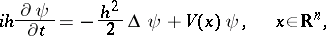(1)

describes the motion of a quantum-mechanical particle in a potential field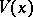. The motion of a classical particle is described by the Hamilton–Jacobi equation (cf. Hamilton–Jacobi theory)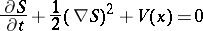(2)

or by the Hamiltonian system(3)

The Cauchy problem for the Schrödinger equation,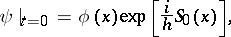(4)

is compared with the Cauchy problem for the system (3):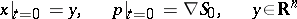(5)

(here the functionsare smooth,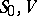are real-valued andis of compact support). The asymptotics of the solution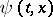as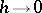,, and for small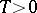have the form:(6)

Here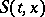is the solution of (2) with Cauchy data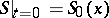(the classical action), while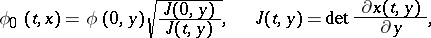where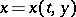,is the solution of (3), (5). The functions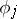for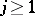are defined from the recurrent system of transport equations (these are ordinary differential equations along the trajectories of the system (3)), so that all terms of the asymptotics are expressed in the terminology of classical mechanics. The Bohr correspondence principle asserts: Iftends to zero, then the quantum laws must go over to the classical laws. The method of seeking asymptotics in the form (6) was proposed by P. Debye and has been widely applied in quantum mechanics.

The asymptotic solution of the problem (1), (4) in the large (that is, for any finite time) is constructed via the canonical operator of V.P. Maslov . The Cauchy data (5) fill out an-dimensional Lagrangian manifold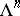in the phase space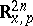. Its translates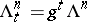along the trajectories of the system (3) are also Lagrangian manifolds; their union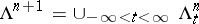is an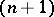-dimensional Lagrangian manifold in the phase spacewith coordinates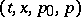. For the canonical operator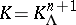corresponding to, the following commutation relation holds: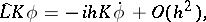(7)

where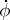is the derivative along the system (3) and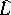is the Schrödinger operator. The asymptotics of the solutionin the large are given by the formula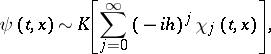(8)

where the functions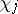are defined from the Cauchy data (4) by means of the transport equations and can be expressed in terms of classical mechanics. At a non-focal pointthe asymptotics have the formwhere the sum is taken over all rays arriving at this point,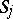and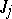are the action and Jacobian for the-th ray andis the Morse index of the-th ray. For the stationary Schrödinger equation in the semi-classical approximation the problem of scattering has been studied, as well as the problem of the field of a point source, and the classical series (of Balmer type) of eigenvalues has been obtained.

Semi-classical approximations in the wide sense (synonyms: high-frequency asymptotics, short-wave approximations, approximations of geometrical optics, WKB-method, eikonal method, quasi-classical approximations in the wide sense) are the asymptotics of solutions of partial differential equations with real characteristics of the form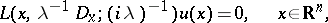(9)

as well as of differential and pseudo-differential equations. Here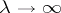is a large parameter and the symboldepends weakly on. Corresponding to (9) are the equations of classical mechanics, namely the Hamilton–Jacobi equationand the Hamiltonian system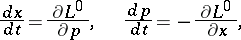(10)

where. The semi-classical approximation is constructed by means of the canonical operator corresponding to the Lagrangian manifolds that are invariant with respect to the dynamical system (10) and having a form similar to (8).

The semi-classical approximation is widely applied in modern physics in problems of the propagation of sound, elastic and electromagnetic waves, in non-relativistic and relativistic quantum mechanics and other questions.

How to Cite This Entry:
Semi-classical approximation. Encyclopedia of Mathematics. URL: http://encyclopediaofmath.org/index.php?title=Semi-classical_approximation&oldid=16814
This article was adapted from an original article by M.V. Fedoryuk (originator), which appeared in Encyclopedia of Mathematics - ISBN 1402006098. See original article